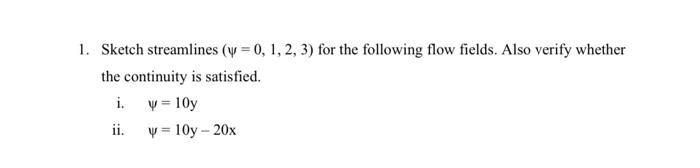Home / Expert Answers / Civil Engineering / solve-this-nbsp-subject-floud-nbsp-sketch-streamlines-0-1-2-3-for-the-following-flow-fi-pa754

# (Solved): Solve this . Subject :floud Sketch streamlines ( = 0, 1, 2, 3) for the following flow fi ...

Solve this .
Subject :floud

Sketch streamlines (? = 0, 1, 2, 3) for the following flow fields. Also verify whether the continuity is satisfied.
i. ? = 10y
ii. ? = 10y – 20x1. Sketch streamlines $$(\psi=0,1,2,3)$$ for the following flow fields. Also verify whether the continuity is satisfied. i. $$\psi=10 \mathrm{y}$$ ii. $$\psi=10 \mathrm{y}-20 \mathrm{x}$$

We have an Answer from Expert